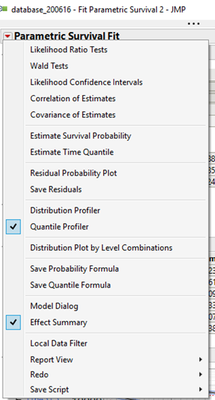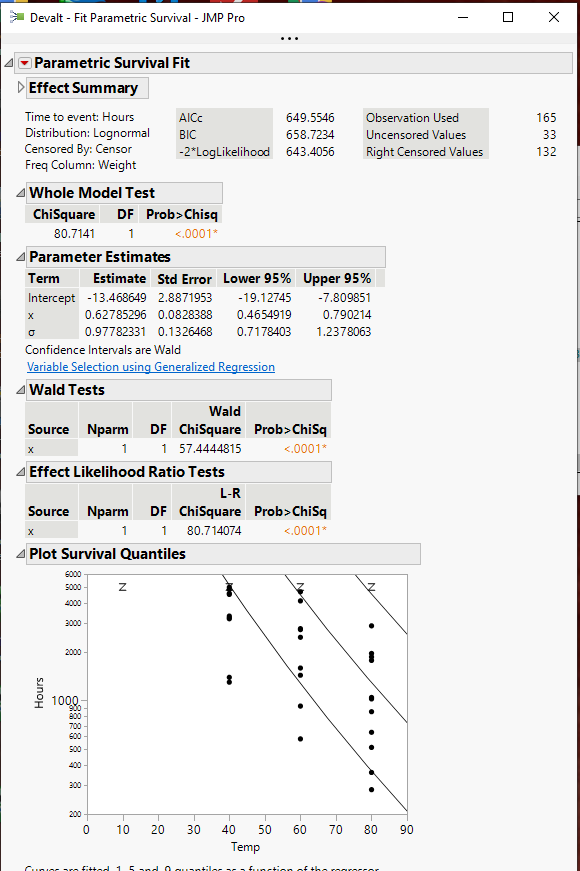Choose Language Hide Translation Bar
Highlighted

## How to find the option "Plot Survival Quantiles" in JMP 15?

Hi,

the manual mentions a "Plot Survival Quantiles"  option for in the Parametric Survival Fit Report, but I can't find it (including in the hidden part of the menu that's accessible through "shift" button).

Is that pot only available in JMP PRO? (It's not indicated as such in the manual.)1 ACCEPTED SOLUTION

Accepted Solutions
Highlighted

## Re: How to find the option "Plot Survival Quantiles" in JMP 15?

When I run the example for Parametric Survival Models from the Statistics Index,

``````Names Default To Here( 1 );
Open(
"\$SAMPLE_DATA/Reliability/Devalt.jmp"
) <<
Run Script(
"Fit Parametric Survival"
);``````

which runs the script from the data table

``````Fit Model(
Freq( :Weight ),
Y( :Hours ),
Effects( :x ),
Personality( "Parametric Survival" ),
Distribution( LogNormal ),
Censor( :Censor ),
Run(
Likelihood Ratio Tests( 1 ),
{Estimate Survival Probability( x = 40.9853, [30000, 10000], Alpha( 0.05 ) )
}
)
)``````

The Plot Survival Quantiles Plot is displayed as part of the default outputJim
3 REPLIES 3
Highlighted

## Re: How to find the option "Plot Survival Quantiles" in JMP 15?

When I run the example for Parametric Survival Models from the Statistics Index,

``````Names Default To Here( 1 );
Open(
"\$SAMPLE_DATA/Reliability/Devalt.jmp"
) <<
Run Script(
"Fit Parametric Survival"
);``````

which runs the script from the data table

``````Fit Model(
Freq( :Weight ),
Y( :Hours ),
Effects( :x ),
Personality( "Parametric Survival" ),
Distribution( LogNormal ),
Censor( :Censor ),
Run(
Likelihood Ratio Tests( 1 ),
{Estimate Survival Probability( x = 40.9853, [30000, 10000], Alpha( 0.05 ) )
}
)
)``````

The Plot Survival Quantiles Plot is displayed as part of the default outputJim
Highlighted

## Re: How to find the option "Plot Survival Quantiles" in JMP 15?

Thanks, after some trials it seems you can only have this plot when there is only a single variable in the model.

Highlighted

## Re: How to find the option "Plot Survival Quantiles" in JMP 15?

Perhaps a profiler would be better suited to the case of multiple predictors.

Learn it once, use it forever!
Article Labels